# RD Sharma Solutions for Class 9 Maths Chapter 7 Introduction to Euclid's Geometry Exercise 7.1

RD Sharma Solutions for Class 9 Maths is given here for chapter 7 – Introduction to Euclid’s Geometry. In this exercise, students will study some geometric basic concepts such as point, line and plane. It is not possible to define these three concepts precisely. However, we have a good idea of these concepts by solving exercise 7.1 in Chapter 7. RD Sharma Class 9 solutions help students to practice several questions rigorously to be able to grasp the concepts thoroughly.

## Download PDF of RD Sharma Solutions for Class 9 Maths Chapter 7 Introduction to Euclid’s Geometry Exercise 7.1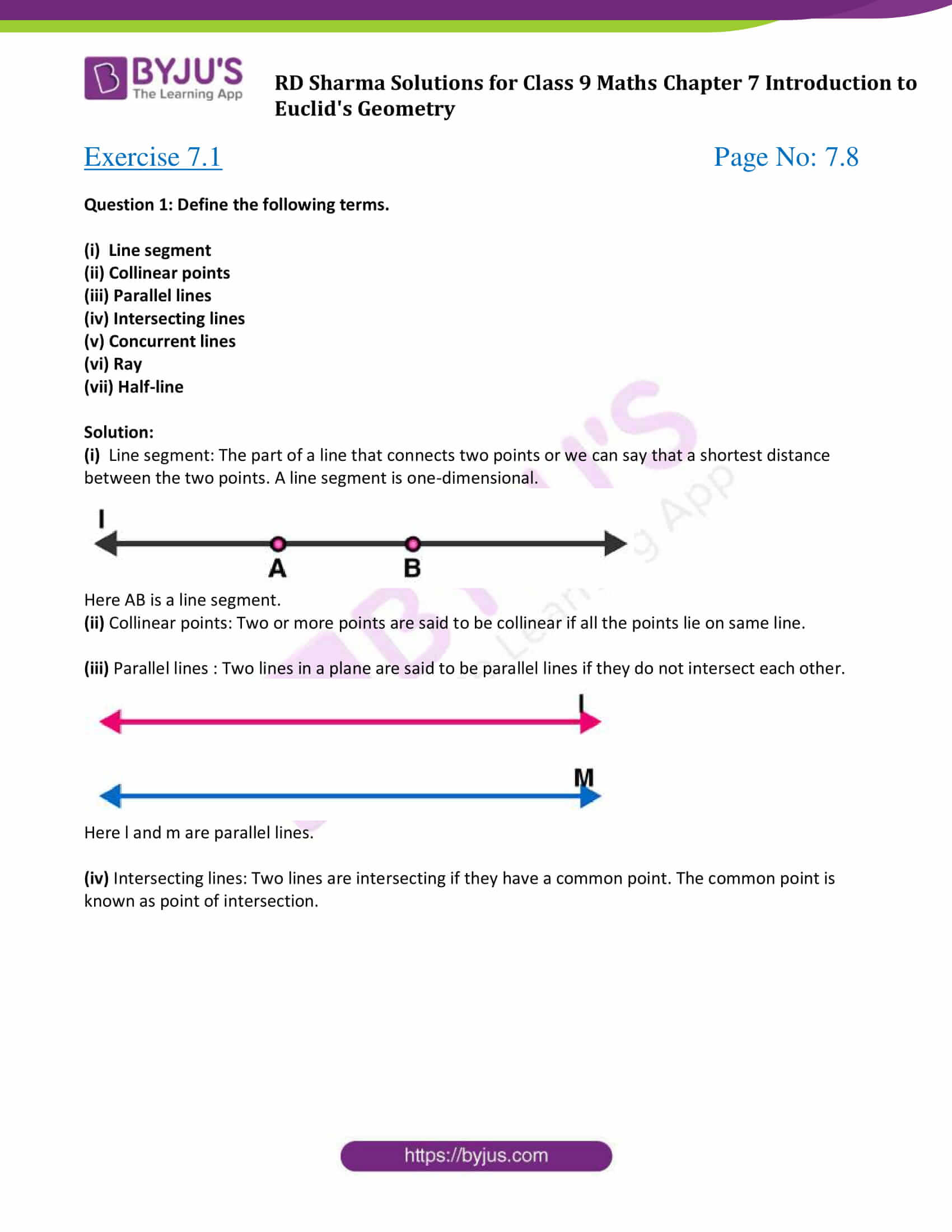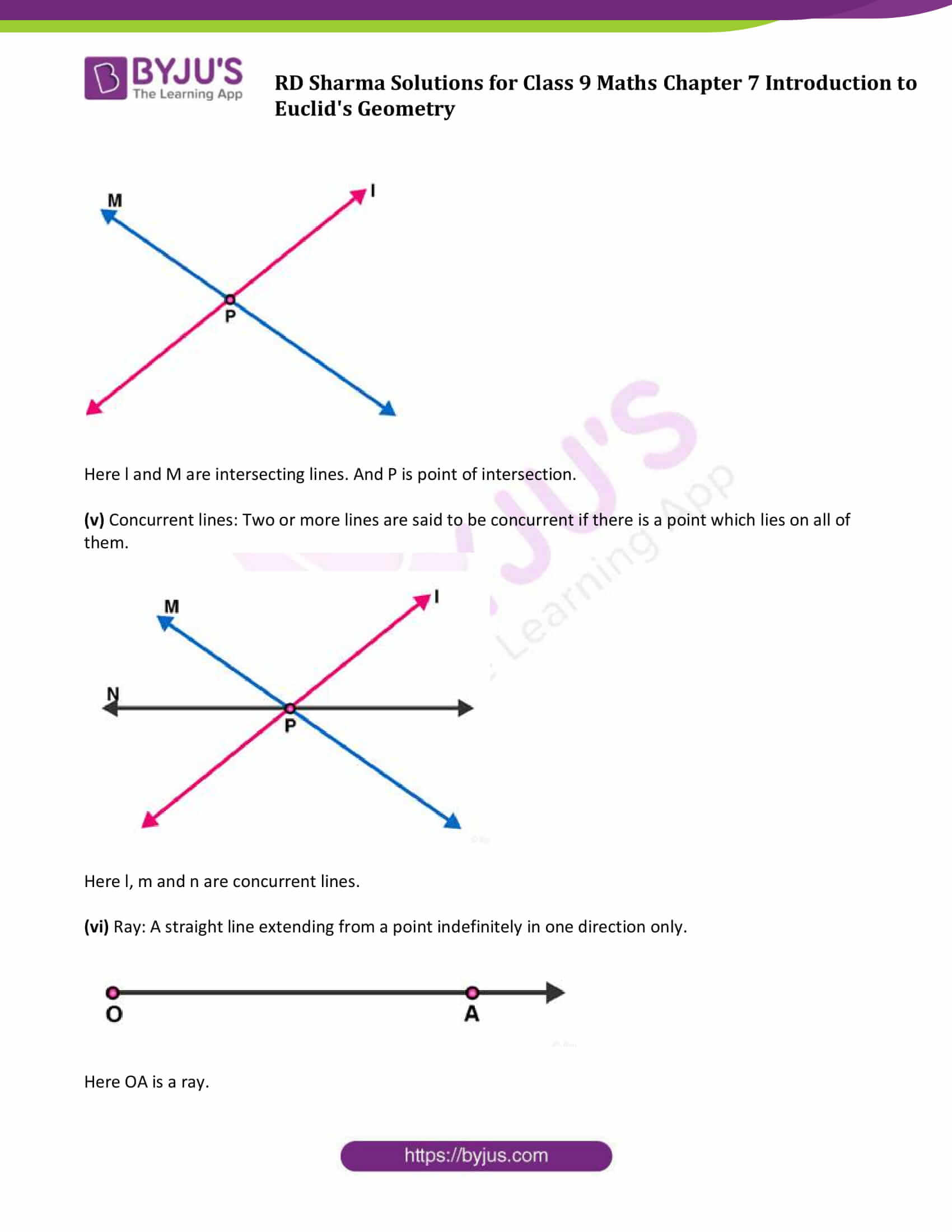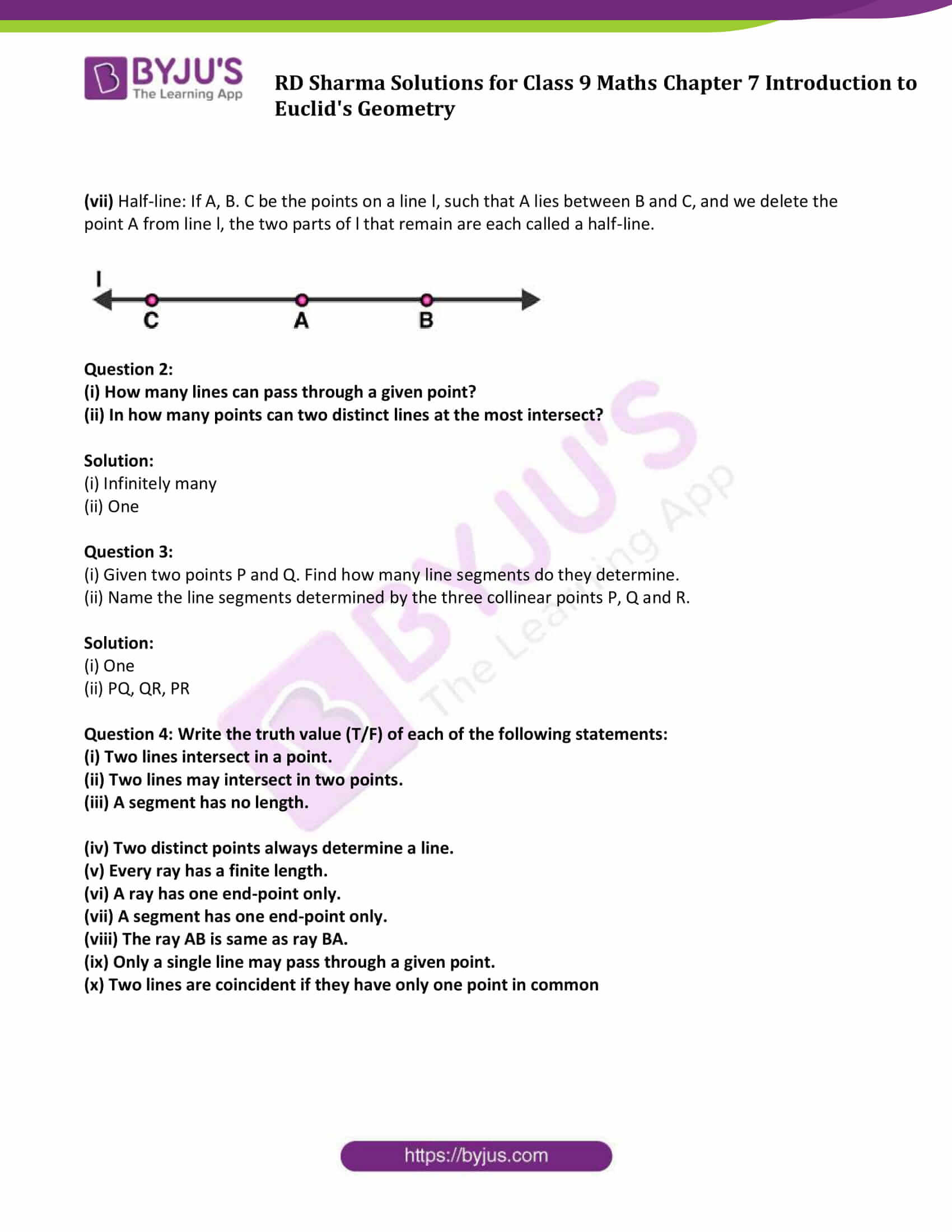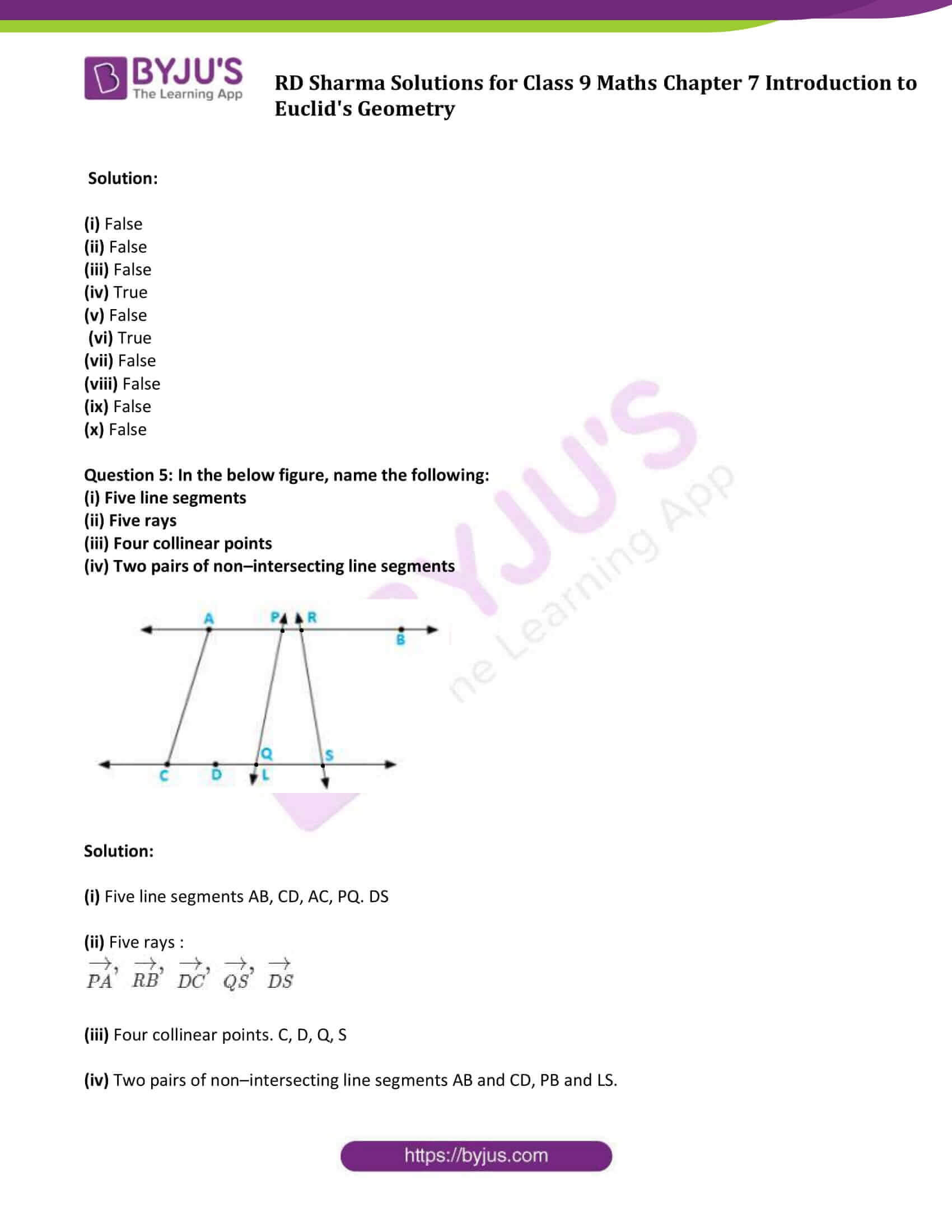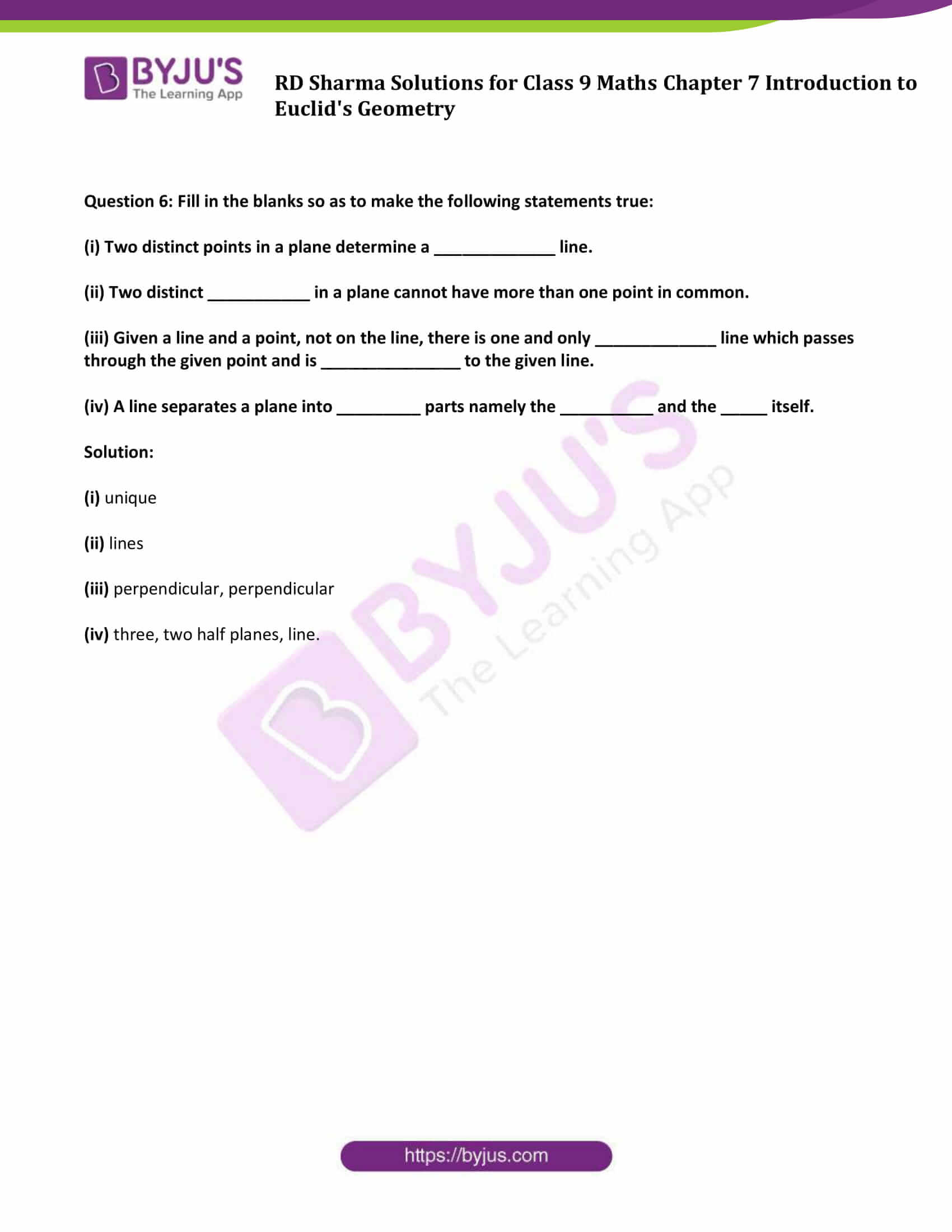### Exercise 7.1 Page No: 7.8

Question 1: Define the following terms.

(i) Line segment

(ii) Collinear points

(iii) Parallel lines

(iv) Intersecting lines

(v) Concurrent lines

(vi) Ray

(vii) Half-line

Solution:

(i) Line segment: The part of a line that connects two points or we can say that a shortest distance between the two points. A line segment is one-dimensional.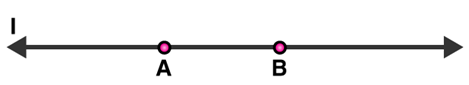Here AB is a line segment.

(ii) Collinear points: Two or more points are said to be collinear if all the points lie on same line.

(iii) Parallel lines : Two lines in a plane are said to be parallel lines if they do not intersect each other.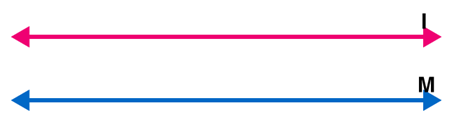Here l and m are parallel lines.

(iv) Intersecting lines: Two lines are intersecting if they have a common point. The common point is known as point of intersection.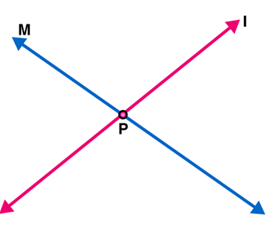Here l and M are intersecting lines. And P is point of intersection.

(v) Concurrent lines: Two or more lines are said to be concurrent if there is a point which lies on all of them.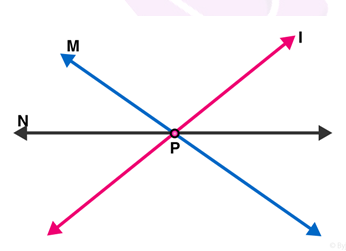Here l, m and n are concurrent lines.

(vi) Ray: A straight line extending from a point indefinitely in one direction only.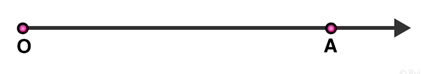Here OA is a ray.

(vii) Half-line: If A, B. C be the points on a line l, such that A lies between B and C, and we delete the point A from line l, the two parts of l that remain are each called a half-line.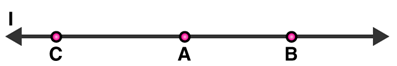Question 2:

(i) How many lines can pass through a given point?

(ii) In how many points can two distinct lines at the most intersect?

Solution:

(i) Infinitely many

(ii) One

Question 3:

(i) Given two points P and Q. Find how many line segments do they determine.

(ii) Name the line segments determined by the three collinear points P, Q and R.

Solution:

(i) One

(ii) PQ, QR, PR

Question 4: Write the truth value (T/F) of each of the following statements:

(i) Two lines intersect in a point.

(ii) Two lines may intersect in two points.

(iii) A segment has no length.

(iv) Two distinct points always determine a line.

(v) Every ray has a finite length.

(vi) A ray has one end-point only.

(vii) A segment has one end-point only.

(viii) The ray AB is same as ray BA.

(ix) Only a single line may pass through a given point.

(x) Two lines are coincident if they have only one point in common

Solution:

(i) False

(ii) False

(iii) False

(iv) True

(v) False

(vi) True

(vii) False

(viii) False

(ix) False

(x) False

Question 5: In the below figure, name the following:

(i) Five line segments

(ii) Five rays

(iii) Four collinear points

(iv) Two pairs of non–intersecting line segments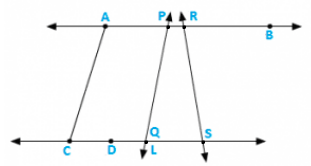Solution:

(i) Five line segments AB, CD, AC, PQ. DS

(ii) Five rays :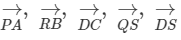(iii) Four collinear points. C, D, Q, S

(iv) Two pairs of non–intersecting line segments AB and CD, PB and LS.

Question 6: Fill in the blanks so as to make the following statements true:

(i) Two distinct points in a plane determine a _____________ line.

(ii) Two distinct ___________ in a plane cannot have more than one point in common.

(iii) Given a line and a point, not on the line, there is one and only _____________ line which passes through the given point and is _______________ to the given line.

(iv) A line separates a plane into _________ parts namely the __________ and the _____ itself.

Solution:

(i) unique

(ii) lines

(iii) perpendicular, perpendicular

(iv) three, two half planes, line.

## RD Sharma Solutions for Class 9 Maths Chapter 7 Introduction to Euclid’s Geometry Exercise 7.1

RD Sharma Solutions Class 9 Maths Chapter 7 Introduction to Euclid’s Geometry Exercise 7.1 is based on the following topics and subtopics:

• Axioms: The Basic facts which are taken for granted, without proof are called axioms.

Some important illustrations are:

-Halves of equal are equal. If a>b and b>c then a>c

-The whole is equal to the sum of its parts and greater than any of its parts.”

• Three basic concepts in geometry, namely, line, point and plane
• Properties of point and lines
• Parallel Lines
• Intersecting Lines
• Line segment
• Interior point of a line segment
• Congruence of line segments
• Length axioms of a line segment
• Line segment length axiom, congruent line segment length axiom, Line segment construction axiom
• Distance between two points
• Mid-point of a line segment
• Ray, Half-line, line separation
• Half-Plane